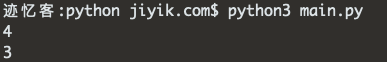# 迹忆客专注技术分享

## 在 Python 中获取浮点数的长度

1. 使用 `str()` 类将浮点数转换为字符串，例如 `result = str(my_float)`
2. 将字符串传递给 `len()` 函数，例如 `len(result)`
3. `len()` 函数将返回字符串的长度。
``````my_float = 3.14

my_str = str(my_float)
print(len(my_str))  # 👉️ 4

without_counting_decimal = len(my_str) - 1
print(without_counting_decimal)  # 👉️ 3
```````len()` 函数返回对象的长度（项目数）。

``````my_float = 3.14

my_str = str(my_float)
print(len(my_str))  # 👉️ 4

no_decimal_1 = len(my_str) - 1
print(no_decimal_1)  # 👉️ 3

no_decimal_2 = len(my_str.replace('.', ''))
print(no_decimal_2)  # 👉️ 3
``````

``````my_float = 3.14

if my_float < 0:
result = len(str(my_float)) - 1
else:
result = len(str(my_float))

print(result)  # 👉️ 4
``````

``````my_float = 3.14

result = len(f'{my_float}')

print(result)  # 👉️ 4
``````

``````my_str = 'is subscribed:'

my_bool = True

result = f'{my_str} {my_bool}'

print(result)  # 👉️ is subscribed: True
``````## 热门标签